Systems of Linear Equations: Graphing (page 2 of 7)

Sections: Definitions, Solving by graphing, Substitition, Elimination/addition, Gaussian elimination.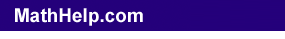When you are solving systems of equations (linear or otherwise), you are, in terms of the equations' related graphed lines, finding any intersection points of those lines.

For two-variable linear systems of equations, there are then three possible types of solutions to the systems, which correspond to three different types of graphs of two straight lines.

These three cases are illustrated below:

 Case 1 Case 2 Case 3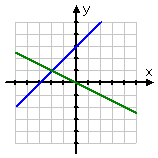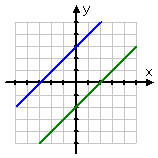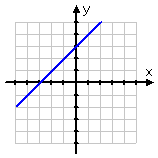The first graph above, "Case 1", shows two distinct non-parallel lines that cross at exactly one point. This is called an "independent" system of equations, and the solution is always some x,y-point.

 Independent system: one solution point Case 2 Case 3The second graph above, "Case 2", shows two distinct lines that are parallel. Since parallel lines never cross, then there can be no intersection; that is, for a system of equations that graphs as parallel lines, there can be no solution. This is called an "inconsistent" system of equations, and it has no solution.

 Independent system: one solution and one intersection point Inconsistent system: no solution and no intersection point Case 3The third graph above, "Case 3", appears to show only one line. Actually, it's the same line drawn twice. These "two" lines, really being the same line, "intersect" at every point along their length. This is called a "dependent" system, and the "solution" is the whole line.

 Independent system: one solution and one intersection point Inconsistent system: no solution and no intersection point Dependent system: the solution is the whole lineThis shows that a system of equations may have one solution (a specific x,y-point), no solution at all, or an infinite solution (being all the solutions to the equation). You will never have a system with two or three solutions; it will always be one, none, or infinitely-many.

Content Continues Below

Probably the first method you'll see for solving systems of equations will be "solving by graphing". Warning: You have to take these problems with a grain of salt. The only way you can find the solution from the graph is IF you draw a very neat axis system, IF you draw very neat lines, IF the solution happens to be a point with nice neat whole-number coordinates, and IF the lines are not close to being parallel.  Copyright © Elizabeth Stapel 2003-2011 All Rights Reserved

 For instance, if the lines cross at a shallow angle it can be just about impossible to tell where the lines cross.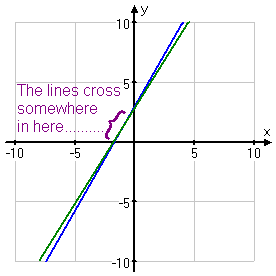And if the intersection point isn't a neat pair of whole numbers, all bets are off.       (Can you tell by looking that the displayed solution has coordinates of (–4.3, –0.95)? No? Then you see my point.)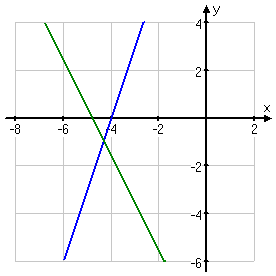On the plus side, since they will be forced to give you nice neat solutions for "solving by graphing" problems, you will be able to get all the right answers as long as you graph very neatly. For instance:

• Solve the following system by graphing.

2x – 3y = –2
4
x +  y = 24

I know I need a neat graph, so I'll grab my ruler and get started. First, I'll solve each equation for "y=", so I can graph easily:

2x – 3y = –2
2x + 2 = 3y
(2/3)x + (2/3) = y

4x + y = 24
y = –4x + 24

The second line will be easy to graph using just the slope and intercept, but I'll need a T-chart for the first line.

 x y = (2/3)x + (2/3) y = –4x + 24 –4 –8/3 + 2/3 = –6/3 = –2 16 + 24 = 40 –1 –2/3 + 2/3 = 0 4 + 24 = 28 2 4/3 + 2/3 = 6/3 = 2 –8 + 24 = 16 5 10/3 + 2/3 = 12/3 = 4 –20 + 24 = 4 8 16/3 + 2/3 = 18/3 = 6 –32 + 24 = –8

(Sometimes you'll notice the intersection right on the T-chart. Do you see the point that is in both equations above? Check the gray-shaded row above.)

 Now that I have some points, I'll grab my ruler and graph neatly, and look for the intersection:                   Even if I hadn't noticed the intersection point in the T-chart, I can certainly see it from the picture.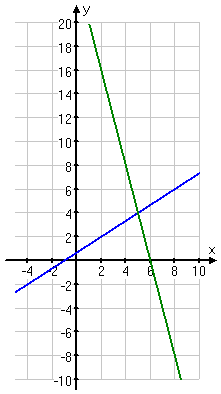solution:  (x, y) = (5, 4)

<< Previous  Top  |  1 | 2 | 3 | 4 | 5 | 6 | 7  |  Return to Index  Next >>

 Cite this article as: Stapel, Elizabeth. "Systems of Linear Equations: Graphing." Purplemath. Available from     https://www.purplemath.com/modules/systlin2.htm. Accessed [Date] [Month] 2016

MathHelp.com Courses

Purplemath:

Study Skills Survey